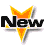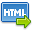Journal :   International Journal of Technology

Volume No. :   6

Issue No. :  2

Year :  2016

Pages :   118-122

ISSN Print :  2231-3907

ISSN Online :  2231-3915Registration

## Linear Stability Analysis of Multicomponent convection

 Address: Jyoti Prakash, Shweta Manan*, Virender Singh Department of Mathematics and Statistics, Himachal Pradesh University, Shimla – 171005 (India) *Corresponding Author
DOI No: 10.5958/2231-3915.2016.00019.5

ABSTRACT:
Condition for characterizing nonoscillatory motions, which may be neutral or unstable, for multicomponent convection is derived. It is analytically proved that the principle of the exchange of stabilities, in multicomponent convection, is valid in the regime (R_1 s)/(2t_1^2 p^4 )+ (R_2 s)/(2t_2^2 p^4 )+?(R_(n-1) s)/(2t_(n-1)^2 p^4 )=1, where R_1,R_2,...,R_(n-1) are the Rayleigh numbers for the (n-1) concentration components and s is the Prandtl number and t_1,t_2,…?,t?_(n-1) are the Lewis numbers for the (n-1) concentration components respectively. When the complement of this sufficient condition holds good, oscillatory motions of neutral or growing amplitude can exist, and thus it is important to derive upper bounds for the complex growth rate of such motions when atleast one of the bounding surfaces is rigid so that exact solutions of the problem in closed form are not obtainable. Thus as a second problem bounds for the growth rates are also obtained. It is further proved that these results are uniformly valid for all combinations of rigid and dynamically free boundaries.
KEYWORDS:
Multicomponent convection, the principle of the exchange of stabilities, oscillatory motions, complex growth rate, concentration Rayleigh number.
Cite:
Jyoti Prakash, Shweta Manan, Virender Singh. Linear Stability Analysis of Multicomponent convection. Int. J. Tech. 2016; 6(2): 118-122.[View HTML][View PDF]

Visitor's No. :   124743